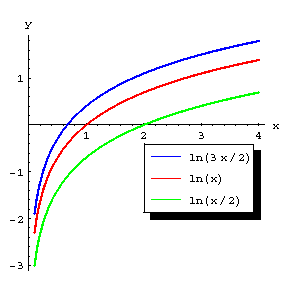# Logarithmic function

logarithm

The function inverse to the exponential function. The logarithmic function is denoted by

$$\tag{1 } y = \mathop{\rm ln} x ;$$

its value $y$, corresponding to the value of the argument $x$, is called the natural logarithm of $x$. From the definition, relation (1) is equivalent to

$$\tag{2 } x = e ^ {y} .$$

Since $e ^ {y} > 0$ for any real $y$, the logarithmic function is defined only for $x > 0$. In a more general sense a logarithmic function is a function

$$y = \mathrm{log} _ {a} x ,$$

where $a > 0$ ($a \neq 1$) is an arbitrary base of the logarithm; this function can be expressed in terms of $\mathop{\rm ln} x$ by the formula

$$\mathrm{log} _ {a} x = \frac{ \mathop{\rm ln} x }{ \mathop{\rm ln} a } .$$

The logarithmic function is one of the main elementary functions; its graph (see Fig.) is called a logarithmic curve.Figure: l060600a

The main properties of the logarithmic function follow from the corresponding properties of the exponential function and logarithms; for example, the logarithmic function satisfies the functional equation

$$\mathop{\rm ln} x + \mathop{\rm ln} y = \mathop{\rm ln} x y .$$

The logarithmic function $y = \mathop{\rm ln} x$ is a strictly-increasing function, and $\lim\limits _ {x \downarrow 0 } \mathop{\rm ln} x = - \infty$, $\lim\limits _ {x \rightarrow \infty } \mathop{\rm ln} x = + \infty$. At every point $x > 0$ the logarithmic function has derivatives of all orders and in a sufficiently small neighbourhood it can be expanded in a power series, that is, it is an analytic function. For $- 1 < x \leq 1$ the following expansion of the (natural) logarithmic function is valid:

$$\mathop{\rm ln} ( 1 + x ) = x - \frac{x ^ {2}}{2} + \frac{x ^ {3}}{3} - \frac{x ^ {4}}{4} + \dots .$$

The derivative of the logarithmic function is

$$( \mathop{\rm ln} x ) ^ \prime = \frac{1}{x} ,\ \ ( \mathrm{log} _ {a} x ) ^ \prime = \ \frac{ \mathrm{log} _ {a} e }{x} = \ \frac{1}{x \mathop{\rm ln} a } .$$

Many integrals can be expressed in terms of the logarithmic function; for example:

$$\int\limits \frac{dx}{x} = \mathop{\rm ln} | x | + C ,$$

$$\int\limits \frac{dx}{\sqrt {x ^ {2} + a } } = \mathop{\rm ln} ( x + \sqrt {x ^ {2} + a } ) + C .$$

The dependence between variable quantities expressed by the logarithmic function was first considered by J. Napier in 1614.

The logarithmic function on the complex plane is an infinitely-valued function, defined for all values of the argument $z \neq 0$, and is denoted by $\mathop{\rm Ln} z$ ( or $\mathop{\rm ln} z$ if no confusion arises). The single-valued branch of this function defined by

$$\mathop{\rm ln} z = \mathop{\rm ln} | z | + i \mathop{\rm arg} z ,$$

where $\mathop{\rm arg} z$ is the principal value of the argument of the complex number $z$, $\pi < \mathop{\rm arg} z \leq \pi$, is called the principal value of the logarithmic function. One has

$$\mathop{\rm Ln} z = \mathop{\rm ln} z + 2 k \pi i ,\ \ k = 0 , \pm 1 ,\dots .$$

All values of the logarithmic function for negative real $z$ are purely imaginary complex numbers. The first satisfactory theory of the logarithmic function for complex arguments was given by L. Euler in 1749; he started from the definition

$$\mathop{\rm Ln} z = \lim\limits _ {n \rightarrow \infty } n ( z ^ {1/n} - 1 ) .$$

How to Cite This Entry:
Logarithmic function. Encyclopedia of Mathematics. URL: http://encyclopediaofmath.org/index.php?title=Logarithmic_function&oldid=51954
This article was adapted from an original article by BSE-3 (originator), which appeared in Encyclopedia of Mathematics - ISBN 1402006098. See original article